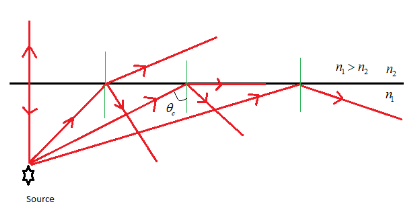QuestionAnswers

# With the help of a ray diagram explain the phenomenon of total internal reflection.Verified
128.7k+ views
Hint: If light passes from optically denser to rarer medium, then at the interface the light is partially reflected back into the denser medium and partially refracted to the rarer medium. This type of reflection is called total internal reflection. When light travels from denser medium to rarer medium at the interface during the refraction the light ray bends away from the normal.

When light passes from denser medium to rarer medium a part of light is reflected back to denser medium and a part of light is refracted to a rarer medium. This phenomenon is called internal reflection. Under certain conditions the whole of the incident light can be made to be reflected back to the denser medium. This is called total internal reflection.As shown in figure when the light ray travels at a small angle of incidence from a denser medium to rarer medium say from water to air, the refracted light bends away from normal so that the angle of refraction is greater than the angle of incidence. As the angle of incidence increases the corresponding angle of refraction also increases. For a certain angle say ${{\theta }_{c}}$ the angle of refraction becomes $90{}^\circ$ i.e. the refracted ray goes along the interface of the two mediums. This angle is called the critical angle. i.e. The critical angle is the angle of incidence when a ray of light travelling from denser to rarer medium after refraction travels along the interface of separation.

If the angle of incidence increased beyond the critical angle ${{\theta }_{c}}$ then no light is refracted into rarer medium (since the angle of refraction cannot be greater than $90{}^\circ$) so the whole of incident ray is reflected back to the denser medium in accordance with the laws of reflection. This phenomenon is known as total internal reflection.

So total internal reflection is defined as the phenomenon in which a ray of light travelling at an angle of incidence greater than the critical angle from denser to a rarer medium is totally reflected back into the denser medium.

Note:
Total internal reflection only occurs when the ray of light is travelling from denser medium to rarer medium i.e. total internal reflection does not occur when the ray travelling from rarer to denser medium. For total internal reflection to occur the angle of incidence must be greater than the critical angle.